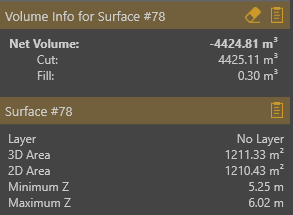Applicable plans
Valley Mountain Peak

The Calculate Volume button is disabled?  Where can I find the measurement? How is the volume calculated? These are common questions and this article explains the basics so that you can start with calculating volumes.

## Required input

Calculate Volume requires a surface item as input:

1. Select a surface
2. The Calculate Volume button becomes enabled
3. You can calculate the volume by clicking on the button.You need to know that:

• When no surface item is selected, the Calculate Volume button is disabled.
• You can also select multiple surfaces and volumes will be calculated for each surface separately.## Calculating the volume

The volume calculation will be done between the current terrain and the selected surface.

• Current terrain means the elevation terrain and the active terrain modifiers (for example after removing a conveyor) that are applied on top of the terrain.
• All available data points will be used in the calculation.
• The volume measurement will become available under the project view (lower left corner) when the surface is selected.## Create a surface

There are several techniques to create a surface for use in volume calculations:

• Using the Quick Surface Tool.
• Triangulate a set of points and lines.
• Import an existing surface from an external CAD or GIS file.

The following video demonstrates a number of use cases where the Quick Surface Tool is used to calculate a volume. Make sure to watch it until the very end to see a more advanced workflow.